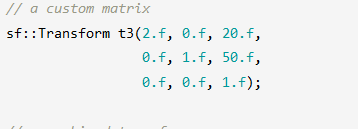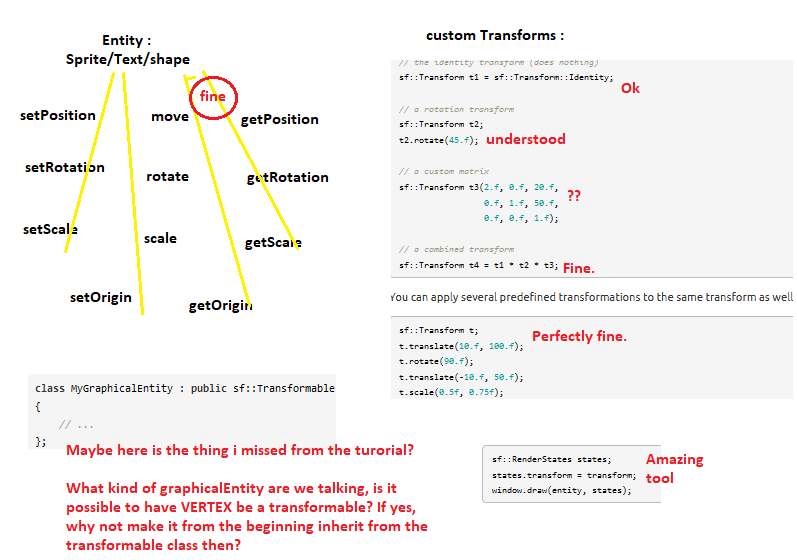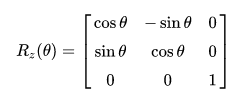###Author Topic: Using ::transform to rotate vertices  (Read 2646 times)

0 Members and 1 Guest are viewing this topic.

#### Power##### Using ::transform to rotate vertices
« on: April 09, 2020, 01:30:55 pm »
Hello,
My first big challenge as a newcomer was to make a concave shape rotate, thanks to A_Sentient_Tomato for the right matrix he shared (https://en.sfml-dev.org/forums/index.php?topic=26984.0), and especially to Hapax for providing this link : https://www.geeksforgeeks.org/2d-transformation-rotation-objects/

https://gfycat.com/fr/realisticwarpedamurstarfish

I used this matrix :
[cos(A)     -sin(A)]
[sin(A)      cos(A)]
Okay, and i know how we got it and what it means
I did not use any transform class , i used only codes of this form :
Quote from: A_Sentient_Tomato
let's say your shape's vertices are {(x,y), (x1, y1), (x2,y2)}
create a new shape with vertices at {(x-C, y-C), (x1-C, y1-C), (x2-C, y2-C)}
_______________________________________________________________
Topic :
Now my problem is this "transform" class thing and its functions https://www.sfml-dev.org/documentation/2.5.1/classsf_1_1Transform.php

____________________________________________________________
It has a 3x3 matrix i saw, here are my questions :

1) How is a 3x3 matrix relevant when we are only working on 2D spaces (sf::window has x and y coordinates only)?

2) is it possible to use the matrix 2x2 that i mentionned (with cos and sin, just above) with the "transform" class?

3) If you have anything to add, or examples about this transform class that is different from what is said on the tutorial, i'd like to hear please.

(My next challenge is to make vertices shapes "expand" in size.....)

Thanks for any enlightenment.
« Last Edit: April 09, 2020, 02:24:54 pm by Power »

#### Laurent

• Hero Member
••• Posts: 32504##### Re: Using ::transform to rotate vertices
« Reply #1 on: April 09, 2020, 07:10:53 pm »
Quote
1) How is a 3x3 matrix relevant when we are only working on 2D spaces (sf::window has x and y coordinates only)?

Quote
2) is it possible to use the matrix 2x2 that i mentionned (with cos and sin, just above) with the "transform" class?
What do you think Transform::rotate does?Quote
3) If you have anything to add, or examples about this transform class that is different from what is said on the tutorial, i'd like to hear please.
If you feel like the documentation or tutorial is lacking important information, I'd like to hear please.

You should really take one or two days to read the documentation and tutorials (and other available resources) carefully. You ask too many questions, I have the feeling that you're not trying enough by yourselfLaurent Gomila - SFML developer

#### Power##### Re: Using ::transform to rotate vertices
« Reply #2 on: April 09, 2020, 10:25:21 pm »
Quote
1) How is a 3x3 matrix relevant when we are only working on 2D spaces (sf::window has x and y coordinates only)?

Quote
2) is it possible to use the matrix 2x2 that i mentionned (with cos and sin, just above) with the "transform" class?
What do you think Transform::rotate does?Quote
3) If you have anything to add, or examples about this transform class that is different from what is said on the tutorial, i'd like to hear please.
If you feel like the documentation or tutorial is lacking important information, I'd like to hear please.

You should really take one or two days to read the documentation and tutorials (and other available resources) carefully. You ask too many questions, I have the feeling that you're not trying enough by yourselfMaybe you are right about me needing to explore this more but i "feel" you are not (i may be wrong), because :
I actually had an answer about a month ago an the subject "do this three features exist?" and i spent whole lot of time RE-READING most tutorials, believe me. Proof i found myself asking if there was a full class diagramm for SFML (you can find it on the suggestions forum), because i was rewrite by hand all the functions i found and try to memorize them.

And ONLY when i suceeded in using the matrix that has been suggested to me a month ago.. i felt "free" to ask my questions. ( you can see that i separated my posts on the graphics forum by weeks). I would not ask this questions if i did not try hard to understand.

Quote
If you feel like the documentation or tutorial is lacking important information, I'd like to hear please.

Yes i feel there is 0 example for the use of a matrix with transform class, i have no idea what the matrix does within the transform and how it works, eventhough i understand where the matrix
[cos(A)     -sin(A)]
[sin(A)      cos(A)]
came from. I know its (NewCoordinaters) = Matrix * (OldCoordinates). So i don't know if i lack more maths maybe. I just don't see what the 3x3 matrix does and i have not encoutenred "any" example using it. Not do i know how to implement the 2x2 matrix above on the class transform.
I managed to write this code :
void pivot(sf::VertexArray &V,float angle,sf::Vertex CenterCoordinates,int NumberOfVertices) // number of points
{
for(int i=0;i<NumberOfVertices;i++)
{

OldPoint_x_shifted=V[i].position.x-CenterCoordinates.position.x;
OldPoint_y_shifted=V[i].position.y-CenterCoordinates.position.y;
NewPoint_x_shifted=COS(angle)*OldPoint_x_shifted-OldPoint_y_shifted*SIN(angle);
NewPoint_y_shifted=OldPoint_x_shifted*SIN(angle)+OldPoint_y_shifted*COS(angle);
V[i].position.x= NewPoint_x_shifted + CenterCoordinates.position.x;  //  NewPoint_x FINAL DESTINATION
V[i].position.y= NewPoint_y_shifted + CenterCoordinates.position.y;  //  NewPoint_y FINAL DESTINATION
cout << "i = " << i <<endl;

}

}
Yet i don't see how to do with the class transform, you should just tell me.
Quote
What do you think Transform::rotate does?
It works for shapes but not for vertices.

Let's take this example from the tutorial  :I still wonder to this day what each part of the 9 points of this matrix does. And how it applies to my (x,y) coordinates for my shape.

This example is perfectly understood :
sf::Transform t;
t.translate(10.f, 100.f);
t.rotate(90.f);
t.translate(-10.f, 50.f);
t.scale(0.5f, 0.75f);
It's perfectly fine, i tested it direclty.

I have no game design previous knowledge nor lot of experience with C++ so the matrix of transform might be for all of you obvious but not for me at least not through reading the turorial.

Let me try to give feedback for the turotial i have read twice :I think i might not know how to do the inheritence from transformable class, nor will i know to use the matrix function anyway. I can't wait to know the answer for my questions and feel stupid and see how easy it was, i am really excited to know the answers.

« Last Edit: April 09, 2020, 10:34:14 pm by Power »

#### Hapax

• Hero Member
••• Posts: 3100
• My number of posts is shown in hexadecimal.##### Re: Using ::transform to rotate vertices
« Reply #3 on: April 09, 2020, 10:40:28 pm »
The matrix is just a standard mathematical matrix and the calculations are standard ones too. Transform does all the things you expect a mathematical matrix to do.

To understand the actual, underlying matrix and how they work, there's a lot of good explanations here:
https://en.wikipedia.org/wiki/Matrix_(mathematics)
(particularly this part: https://en.wikipedia.org/wiki/Matrix_(mathematics)#Linear_transformations )
https://en.wikipedia.org/wiki/Square_matrix

EDIT: fixed broken link (the bracket at the end of the URL was discarded)
« Last Edit: April 09, 2020, 10:52:26 pm by Hapax »
Selba Ward - SFML drawables
Kairos - Timing Library
Rectangular Boundary Collision - Rectangular SAT Collision

#### Power##### Re: Using ::transform to rotate vertices
« Reply #4 on: April 09, 2020, 10:49:22 pm »
The matrix is just a standard mathematical matrix and the calculations are standard ones too. Transform does all the things you expect a mathematical matrix to do.

To understand the actual, underlying matrix and how they work, there's a lot of good explanations here:
https://en.wikipedia.org/wiki/Matrix_(mathematics)
(particularly this part: https://en.wikipedia.org/wiki/Matrix_(mathematics)#Linear_transformations )
https://en.wikipedia.org/wiki/Square_matrix
So my question here, to use this :
[cos(A)     -sin(A)]
[sin(A)      cos(A)]

I will be doing something like this?
#define SIN(x) sin(x * 3.141592653589/180)
#define COS(x) cos(x * 3.141592653589/180)
...
int main()
{

// defining a random shape
sf::RectangulareShape ...
..
float A=25.

sf::Transform t(COS(A) -SIN(A) 0,
SIN(A), COS(A), 0,
0, 0, 0);

...
..
window.draw(shape,transform)

?
I still don't see how it is used. (I know where the matrix with cos and sin came from, i spent few time writing on a paper and understanding it btw)

I just don't see where the sf::transform intervenes, sincerely sorry with my lack on understanding for this specific point.
« Last Edit: April 09, 2020, 10:52:51 pm by Power »

#### Hapax

• Hero Member
••• Posts: 3100
• My number of posts is shown in hexadecimal.##### Re: Using ::transform to rotate vertices
« Reply #5 on: April 09, 2020, 10:54:26 pm »
Ah, more detail about that is here:
https://en.wikipedia.org/wiki/Rotation_matrix

Scroll down to "In three dimensions".

Rotating in 2D is the same as rotating in 3D around the Z axis.
Selba Ward - SFML drawables
Kairos - Timing Library
Rectangular Boundary Collision - Rectangular SAT Collision

#### Power##### Re: Using ::transform to rotate vertices
« Reply #6 on: April 09, 2020, 11:08:19 pm »
Ah, more detail about that is here:
https://en.wikipedia.org/wiki/Rotation_matrix

Scroll down to "In three dimensions".

Rotating in 2D is the same as rotating in 3D around the Z axis.
Aaah Finally thank good! It's been a month i am trying to do this. Now i get it thank youu. The answer was :@Laurent, nevermind you were right, i just wasn't familiar with rotation matrix a lot in my life recently i guess. You would add just a small, few lines to know what could be inside "mygraphicalEntity" maybe.

The last thing i did not fully understand from the transormations tutorial is how to inherit vertices from transformable class and use these functions. I will probably figure it when analysing the game examples SFML provide.

Thanks again!
« Last Edit: April 09, 2020, 11:22:17 pm by Power »

#### Power##### Re: Using ::transform to rotate vertices
« Reply #7 on: April 10, 2020, 10:15:25 am »

Quote
What do you think Transform::rotate does?
It works for shapes but not for vertices.

So i have to find how to use rotate with vertices?
I have to create a class that inherit from transform class, and for every vertix i would be able to apply the rotate thing.. something like that?

#### Laurent

• Hero Member
••• Posts: 32504##### Re: Using ::transform to rotate vertices
« Reply #8 on: April 10, 2020, 10:39:15 am »
Ok, I'll try to summarize:

1. sf::Transform is meant to be a wrapper around a mathematical matrix, so in most cases you don't have to know about matrices, nor about what sf::Transform does internally. Don't use the constructor that takes 9 matrix elements if you don't know what to do with it, just ignore (you can assume it's for "advanced" uses).

2. So, to build your custom transformation, create a sf::Transform instance and translate/rotate/scale it however you like.

3. Then, from it, you can either:
- transform full entites at draw time (ie. it's the GPU that does the job), using sf::RenderStates
- transform single points, using sf::Transform::transformPoint

4. If you want to transform a vertex (it's position), then just write v.position = transform.transformPoint(v.position). But if it's for rendering, use sf::RenderStates instead, when you draw the entity. Transforming points explicitly is rather for computations (collisions, etc.).

Don't forget to mention what is the problem that you're actually trying to solve (what are you trying to transform? for what purpose?), because instead of focusing on matrices we may have more direct answers to your real problemLaurent Gomila - SFML developer

#### Power##### Re: Using ::transform to rotate vertices
« Reply #9 on: April 11, 2020, 12:16:21 am »
Quote
1. sf::Transform is meant to be a wrapper around a mathematical matrix, so in most cases you don't have to know about matrices, nor about what sf::Transform does internally. Don't use the constructor that takes 9 matrix elements if you don't know what to do with it, just ignore (you can assume it's for "advanced" uses).

2. So, to build your custom transformation, create a sf::Transform instance and translate/rotate/scale it however you like.

3. Then, from it, you can either:
- transform full entites at draw time (ie. it's the GPU that does the job), using sf::RenderStates
Alright,
Quote
- transform single points, using sf::Transform::transformPoint
Interesting, this one i missed, i will explore it next on my to SFML to learn list.
Quote
4. If you want to transform a vertex (it's position), then just write v.position = transform.transformPoint(v.position). But if it's for rendering, use sf::RenderStates instead, when you draw the entity. Transforming points explicitly is rather for computations (collisions, etc.)
Noted, good to know.
Quote
Don't forget to mention what is the problem that you're actually trying to solve (what are you trying to transform? for what purpose?), because instead of focusing on matrices we may have more direct answers to your real problem
Alright, thank you.